# 【智能优化算法-鲸鱼算法】基于鲸鱼算法求解多目标优化问题附matlab代码(NSWOA)

+关注继续查看

## ?1 内容介绍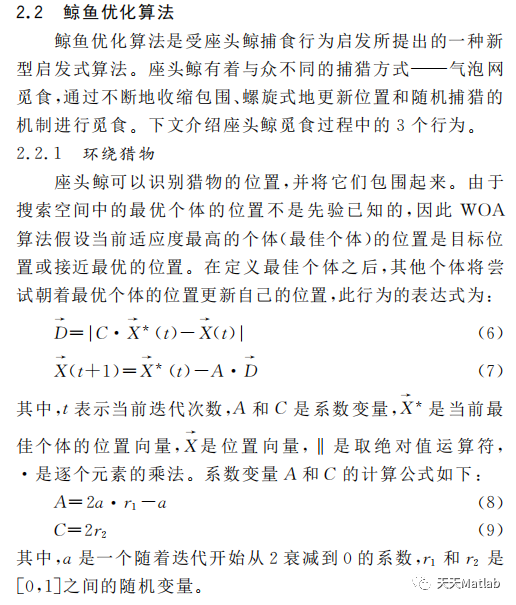编辑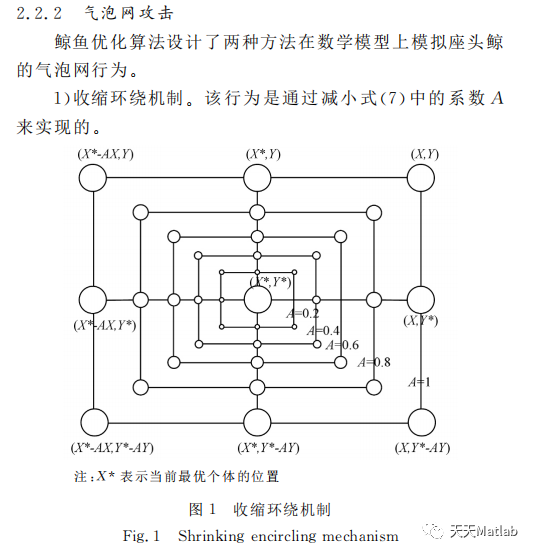编辑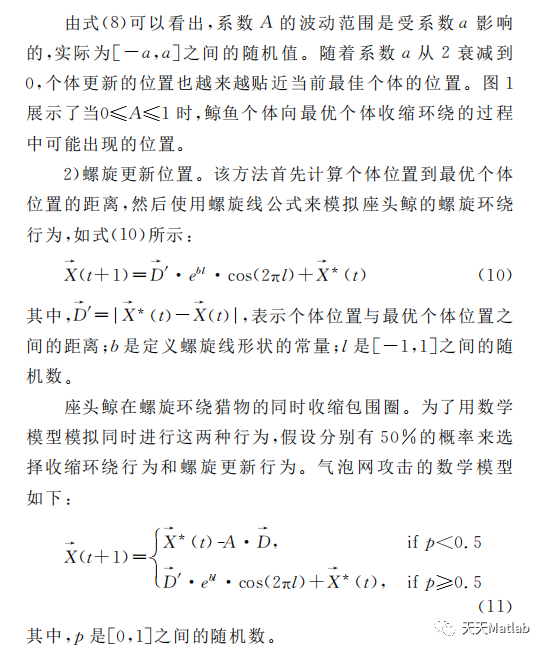编辑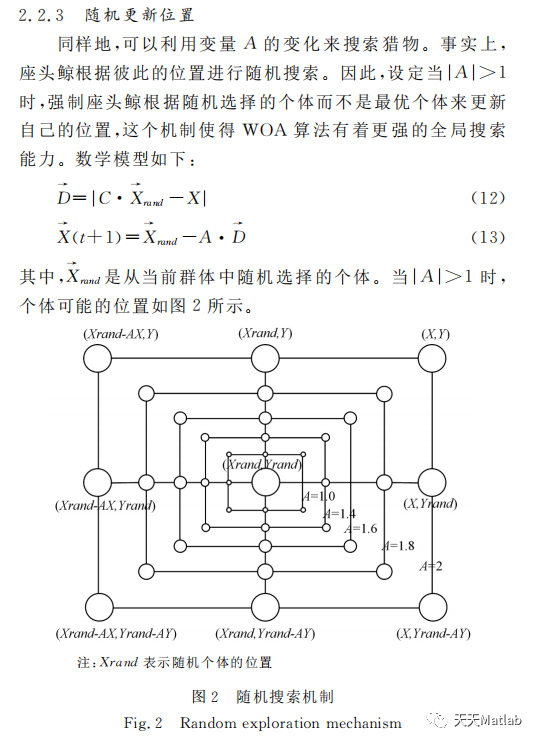编辑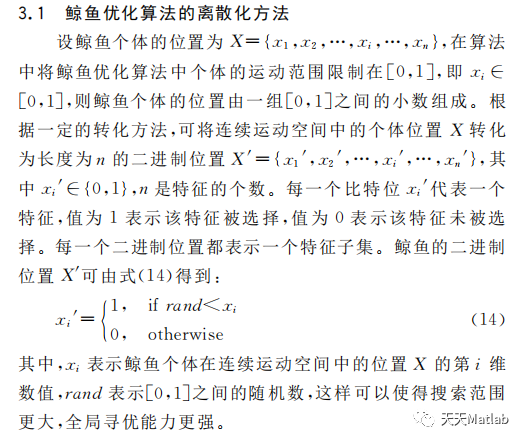编辑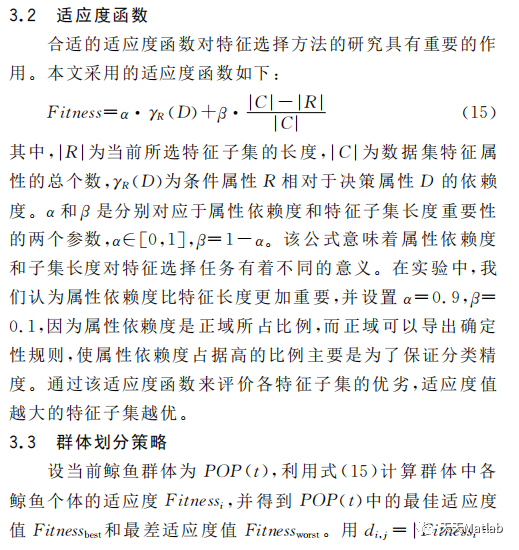编辑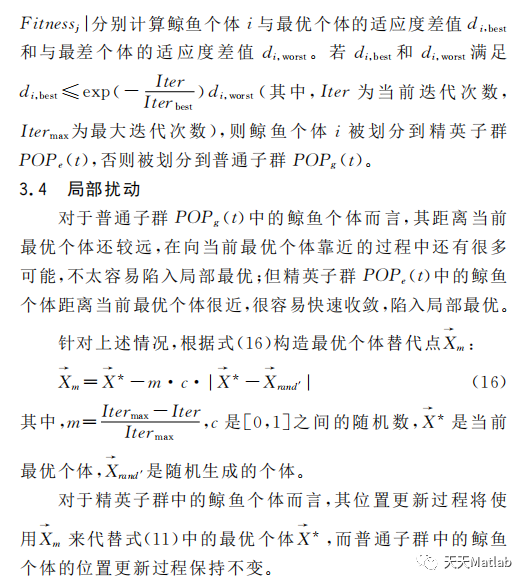编辑

## 2 仿真代码

%%?Non Sorted Whale Optimization Algorithm?(NSWOA)

% NSWOA is developed by Pradeep Jangir

%% Objective Function

% The objective function description contains information about the

% objective function. M is the dimension of the objective space, D is the

% dimension of decision variable space, LB and UB are the

% range for the variables in the decision variable space. User has to

% define the objective functions using the decision variables. Make sure to

% edit the function 'evaluate_objective' to suit your needs.

clc

clear all

D = 30; % Number of decision variables

M = 2; % Number of objective functions

K=M+D;

LB = ones(1, D).*0; %? LB - A vector of decimal values which indicate the minimum value for each decision variable.

UB = ones(1, D).*1; % UB - Vector of maximum possible values for decision variables.

Max_iteration = 100;? % Set the maximum number of generation (GEN)

SearchAgents_no = 100;? ? ? % Set the population size (Search Agent)

ishow = 10;

%% Initialize the population

% Population is initialized with random values which are within the

% specified range. Each chromosome consists of the decision variables. Also

% the value of the objective functions, rank and crowding distance

% information is also added to the chromosome vector but only the elements

% of the vector which has the decision variables are operated upon to

% perform the genetic operations like corssover and mutation.

chromosome = initialize_variables(SearchAgents_no, M, D, LB, UB);

%% Sort the initialized population

% Sort the population using non-domination-sort. This returns two columns

% for each individual which are the rank and the crowding distance

% corresponding to their position in the front they belong. At this stage

% the rank and the crowding distance for each chromosome is added to the

% chromosome vector for easy of computation.

intermediate_chromosome = non_domination_sort_mod(chromosome, M, D);

%% Perform Selection

% Once the intermediate population is sorted only the best solution is

% selected based on it rank and crowding distance. Each front is filled in

% ascending order until the addition of population size is reached. The

% last front is included in the population based on the individuals with

% least crowding distance

% Select NP fittest solutions using non dominated and crowding distance

% sorting and store in population

Population = replace_chromosome(intermediate_chromosome, M,D,SearchAgents_no);

%% Start the evolution process

% The following are performed in each generation

% * Select the parents which are fit for reproduction

% * Perfrom crossover and Mutation operator on the selected parents

% * Perform Selection from the parents and the offsprings

% * Replace the unfit individuals with the fit individuals to maintain a

%? ?constant population size.

Pareto = NSWOA(D,M,LB,UB,Population,SearchAgents_no,Max_iteration,ishow);

save Pareto.txt Pareto -ascii;? % save data for future use

%% Plot data

if M == 2

? ? plot_data2(M,D,Pareto)

elseif M == 3

? ? plot_data_TCQ(M,D,Pareto);?

end

## 3 运行结果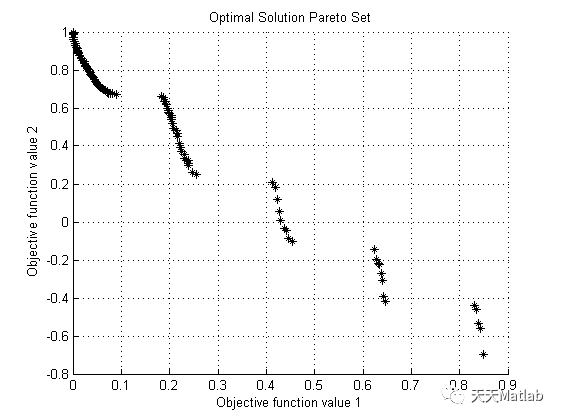编辑

## 4 参考文献

滕德云, 滕欢, 刘鑫,等. 基于改进鲸鱼优化算法的多目标无功优化调度[J]. 电力电容器与无功补偿, 2019, 40(3):7.

梁倩. 基于反向精英保留和Levy变异的多目标鲸鱼优化算法[J]. 现代计算机, 2021(18):7.

### 博主简介：擅长智能优化算法、神经网络预测、信号处理、元胞自动机、图像处理、路径规划、无人机等多种领域的Matlab仿真，相关matlab代码问题可私信交流。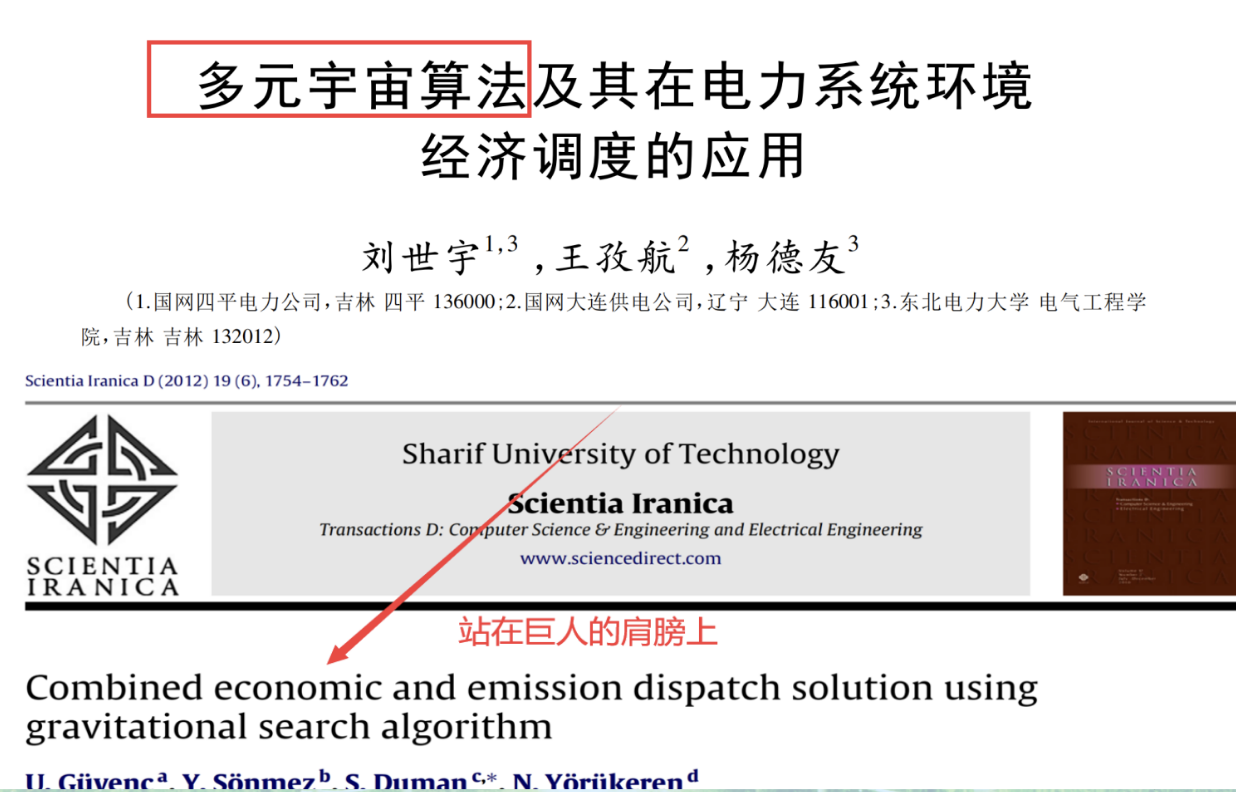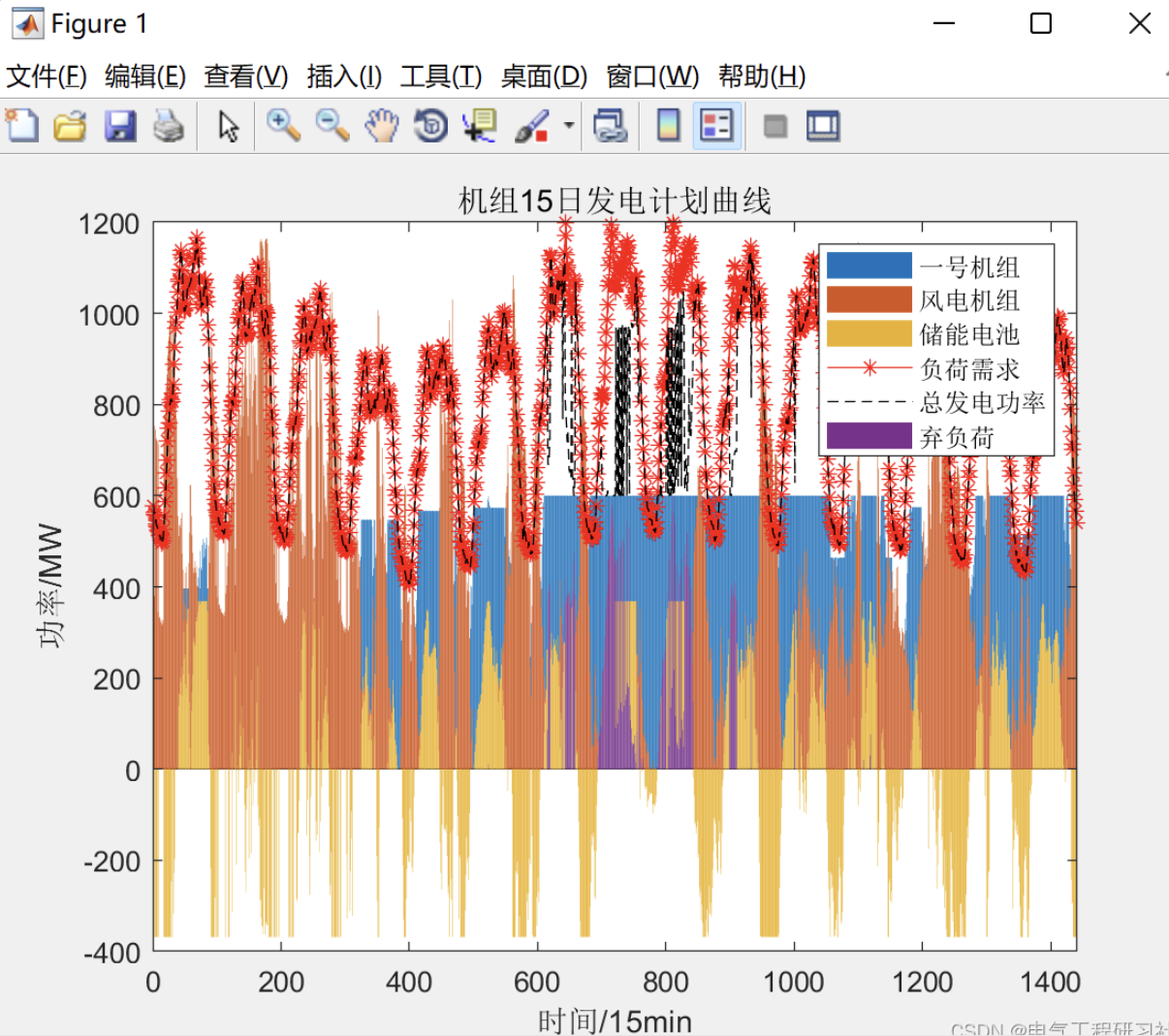【电力系统经济调度】多元宇宙算法求解电力系统多目标优化问题（Matlab实现）【电气期刊论文复现】
【电力系统经济调度】多元宇宙算法求解电力系统多目标优化问题（Matlab实现）【电气期刊论文复现】
0 0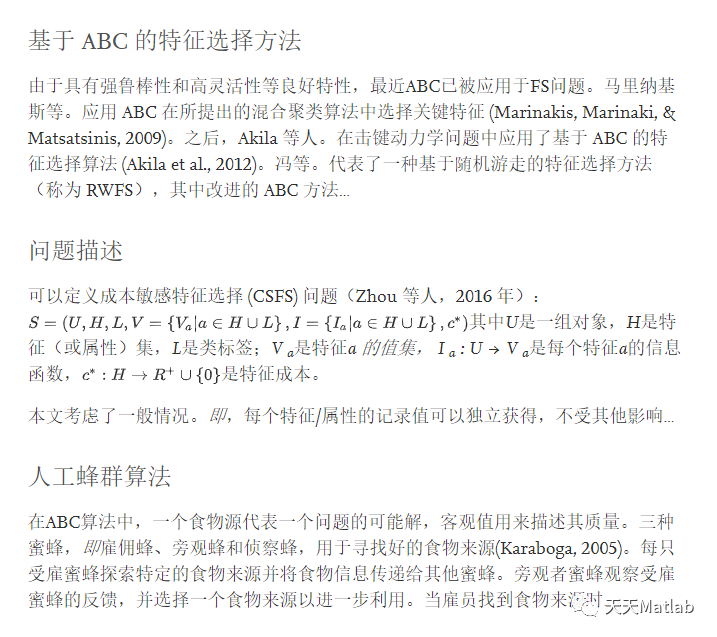0 0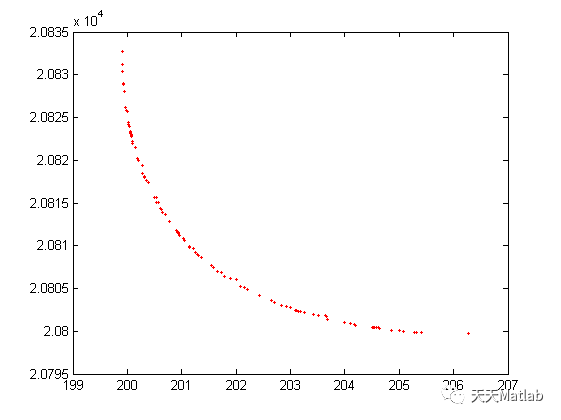【电力系统经济调度】多元宇宙算法求解电力系统多目标优化问题附Matlab
【电力系统经济调度】多元宇宙算法求解电力系统多目标优化问题附Matlab
0 0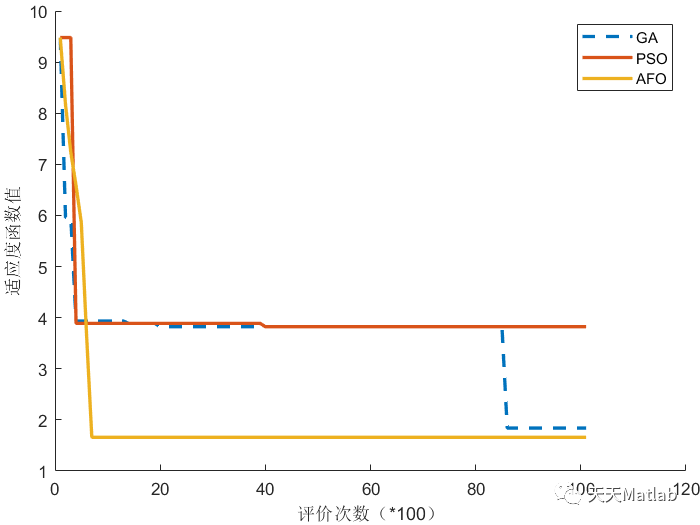【多式联运】基于帝企鹅AFO 粒子群PSO 遗传GA算法求解不确定多式联运路径优化问题附matlab代码
【多式联运】基于帝企鹅AFO 粒子群PSO 遗传GA算法求解不确定多式联运路径优化问题附matlab代码
0 0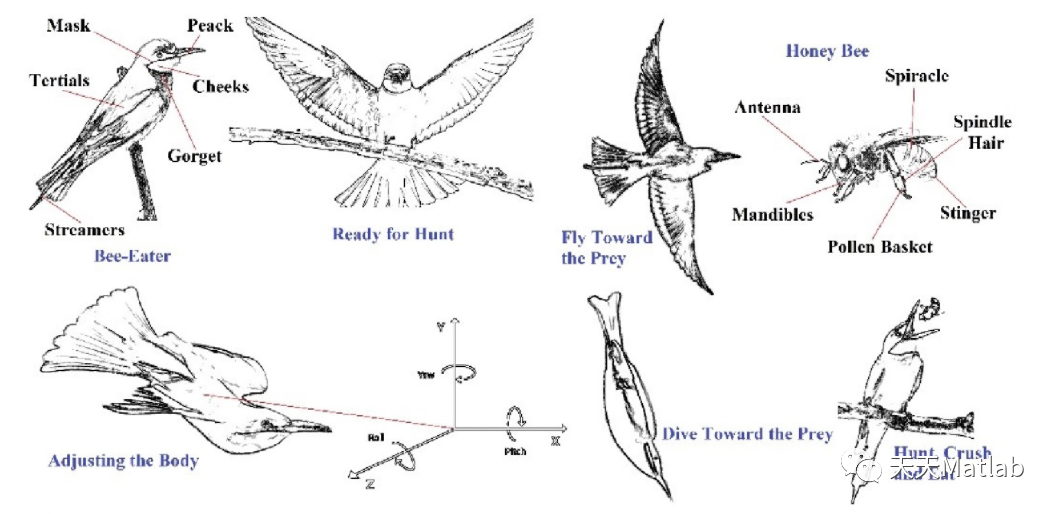0 0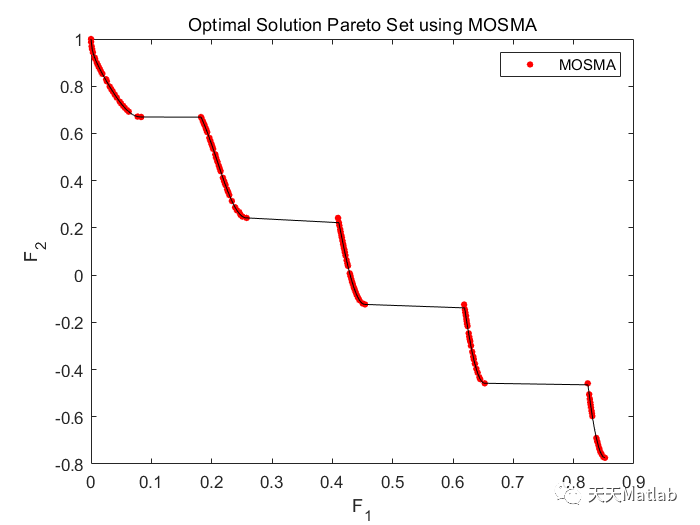【MOSMA】基于粘菌算法求解多目标优化问题附matlab代码
【MOSMA】基于粘菌算法求解多目标优化问题附matlab代码
0 0【算法系列】凸优化的应用——Python求解优化问题（附代码）
【算法系列】凸优化的应用——Python求解优化问题（附代码）
0 0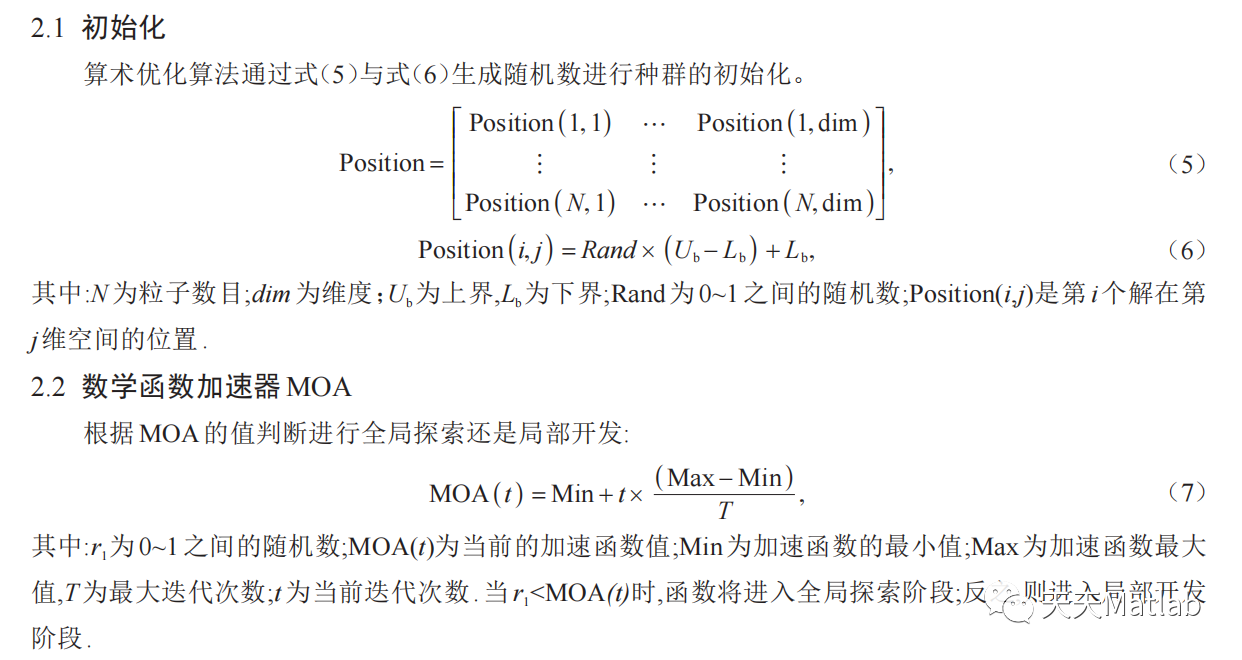【智能优化算法-算术算法】基于算术优化算法 (MAOA)求解多目标优化问题附matlab代码
【智能优化算法-算术算法】基于算术优化算法 (MAOA)求解多目标优化问题附matlab代码
0 0
Matlab科研工作室

【算法实战】5. Logistic回归算法376

【算法实战】2. K近邻算法1255

【算法实战】6. 支持向量机算法491070613

【算法实战】9. 线性回归算法275

【算法实战】4. 朴素贝叶斯算法976